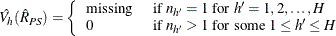#### Poststratification

After a probability sample is drawn and survey data are collected, researchers sometimes want to stratify the sample according to auxiliary information about the sampled population. This process is often called poststratification.

When poststratification is done properly, it can improve efficiency. It can also be used to adjust the sampling weights such that the marginal distribution of the sampling weights is in agreement with known auxiliary information from other resources, such as the census. The adjusted weight is often called the poststratification weight. It is quite common for researchers to use poststratification techniques in survey data analysis.

Poststratification is also used by epidemiologists, who frequently analyze health survey data. They often compute statistics based on a process called direct standardization, a form of poststratification. For example, certain diseases, such as cancer, are more common among older populations. Therefore, to compare the prevalence rates among geographic regions that are populated with different age groups, it is necessary to make adjustments according to such demographic categories and to compute relative prevalence rates of the diseases.

For more information about poststratification, see Fuller (2009); Lohr (2010); Wolter (2007); Rao, Yung, and Hidiroglou (2002).

After you provide the population controls for each poststratum that is defined by the poststratification variables, the SURVEYMEANS procedure creates the poststratification weights accordingly. Then the procedure computes statistics that you request by using poststratification weights.

You can save the poststratification weights in an OUTPSWGT= data to be used in subsequent analyses.

For a select sample, let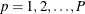be the poststratum index; let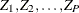be the population totals for each corresponding poststratum; and let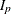be a corresponding indicator variable for the poststratum p defined by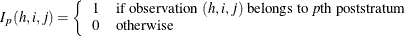Denote the total sum of original weights in the sample for each poststratum as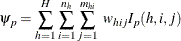Then the poststratification weight for the observation (h, i, j) is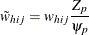The SURVEYMEANS procedure computes statistics by using the poststratification weights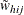instead of the original weights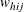.

The standard error and confidence intervals of computed statistics are based on the estimated variance, using either a replication method or the Taylor series method.

##### Replication Methods

When you specify VARMETHOD=BRR or VARMETHOD=JACKKNIFE, PROC SURVEYMEANS computes the variance of a statistic by using replication methods, as described in the section Replication Methods for Variance Estimation. However, with poststratification, an extra step is needed to adjust the weights.

First, PROC SURVEYMEANS constructs a replicate and computes appropriate replicate weights for the replicate. Then, by using the poststratification control totals, the procedure adjusts these replicate weights in the same way as described previously for constructing the poststratification weights for the full sample. Finally, PROC SURVEYMEANS computes the estimate for a desired statistics by using the poststratification weights that are adjusted from the replicate weights in the current replicate. Then the final variance is estimated by the variability among replicate estimates, as described in the section Replication Methods for Variance Estimation.

##### Taylor Series Method

When you specify VARMETHOD=TAYLOR, or by default when you do not specify the VARMETHOD= option, PROC SURVEYMEANS uses the Taylor series method to estimate the variances of requested statistics.

###### Variance of the Mean

The mean of variable Y under poststratification is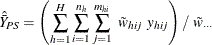where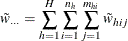is the sum of the poststratification weights over all observations in the sample.

For each poststratum, let the mean of variable Y in each poststratum be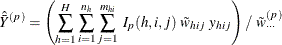where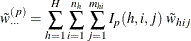is the total of the poststratification weights in poststratum p.

For observation (h, i, j), let p be the poststratum to which it belongs. Let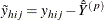PROC SURVEYMEANS estimates the variance of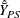as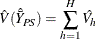where, if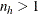, then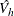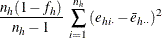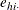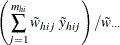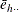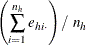and if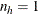, then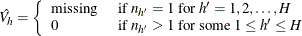###### Variance of the Ratio

Suppose you want to calculate the ratio of variable Y to variable X. Let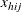and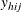be the values of variable X and variable Y, respectively, for observation (h, i, j).

The ratio of Y to X after poststratification is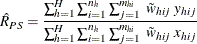whereis the poststratification weight for observation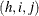.

Let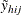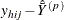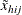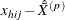where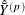and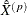are the means of variable Y and variable X, respectively, in poststratum p.

The variance of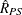is estimated by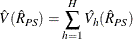where, if, then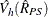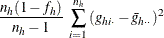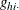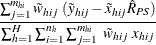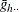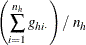and if, then